# Separate Text & Numbers – Excel & Google Sheets

This tutorial will demonstrate you how to separate text and numbers from an alphanumerical string in Excel and Google Sheets.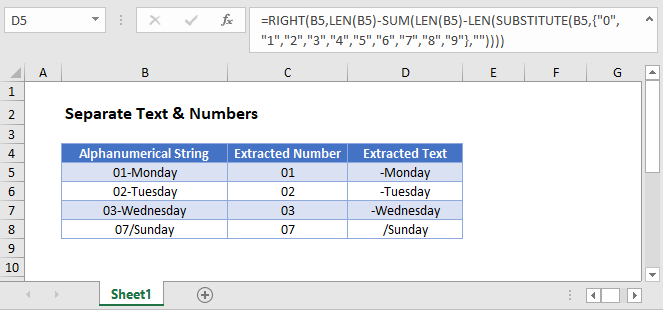## Separate Number and Text from String

This article will discuss how to split numbers and text if you have alphanumerical data where the first part is text and the last part is numerical (or vis-a-versa).you need only the number part from. For more complex cases see How to Remove Non-Numeric Character article.

## Extract Number from the Right

The easiest case of extracting numbers from a string is when the number can be found at the right end of that string. First we locate the starting position of the number with the FIND function and then extract it with the RIGHT function.

``=RIGHT(B3,LEN(B3)-MIN(FIND({0,1,2,3,4,5,6,7,8,9},B3&"0123456789"))+1)``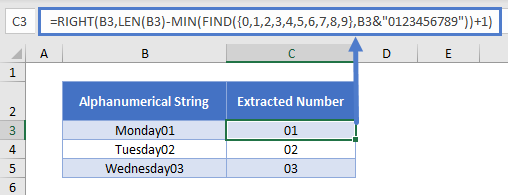Let’s go through the above formula.

### Find First Number

We can use the FIND function to locate the starting position of the number.

``=MIN(FIND({0,1,2,3,4,5,6,7,8,9},B3&"0123456789"))``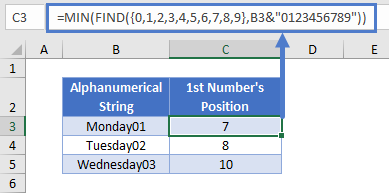For the find_text argument of the FIND function, we use the array constant {0,1,2,3,4,5,6,7,8,9}, which makes the FIND function perform separate searches for each value in the array constant.

The within_text argument of the FIND function is cell value & “0123456789”. In our example, “Monday010123456789”.

Since the array constant contains 10 numbers, the result will be an array of 10 values. In our example: {7,8,11,12,13,14,15,16,17,18}. Then we simply look for the minimum of number positions within this array and get therefore the place of the first number.

### Extract Number Part

Once we have the starting position of the number found at the end of our alphanumerical string, we can use the RIGHT function to extract it.

``=RIGHT(B3,LEN(B3)-C3+1)``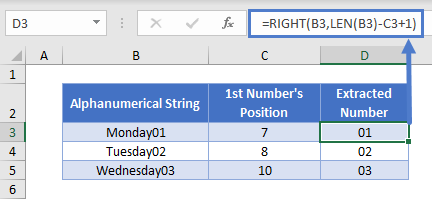### Extract Text Part

With the starting position of the number part we can determine the end of the text part at the same time. We can use the LEFT function to extract it.

``=LEFT(B3,C3-1)``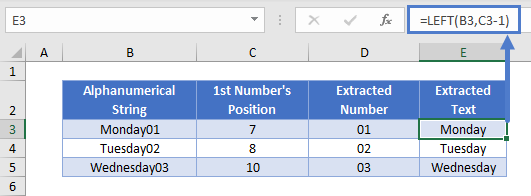# Extract Number from the Left

A more complicated case of extracting numbers from a string is when the number can be found at the beginning (i.e., left side) of the string. Obviously, you don’t need to find its starting position, rather the position where it ends. First we find the last number’s position with the help of the SUBSTITUTE function and then extract the number with the LEFT function.

``=LEFT(B3,SUM(LEN(B3)-LEN(SUBSTITUTE(B3,{"0","1","2","3","4","5","6","7","8","9"},""))))``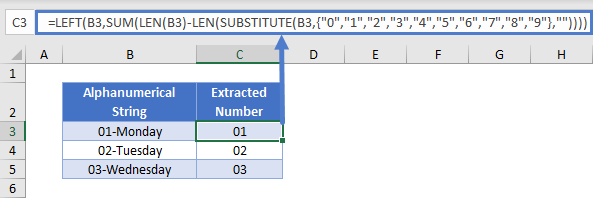Let’s go through the above formula.

### Find Last Number

With the SUBSTITUTE function you can replace every number one by one with an empty string and then sum up how many times you had to do so.

``=SUM(LEN(B3)-LEN(SUBSTITUTE(B3,{"0","1","2","3","4","5","6","7","8","9"},"")))``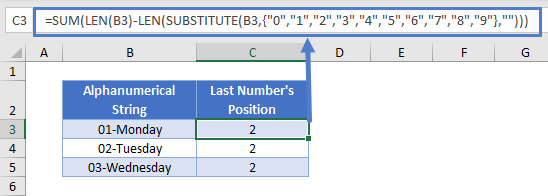When you substitute each number one by one with an empty string, you get every time a string the length of which is one less the original length. In our case the length of 1-Monday and 0-Monday is both 8. Subtracting this length from the original length (9 in our case), you always get 1. When you sum up these, you get the position of your last number.

### Easy Excel Automation### Extract Number Part

Once we have the last position of the number found at the beginning of our alphanumerical string, we can use the LEFT function to extract it.

``=LEFT(B3,C3)``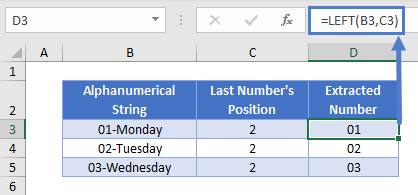### Extract Text Part

Having the last position of the number found at the beginning of our alphanumerical string, we already have the starting position of our text part and can use the RIGHT function to extract it.

``=RIGHT(B3,LEN(B3)-C3)``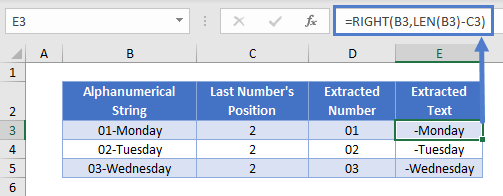## Separate Text & Numbers in Google Sheets

All the examples explained above work the same in Google sheets as they do in Excel.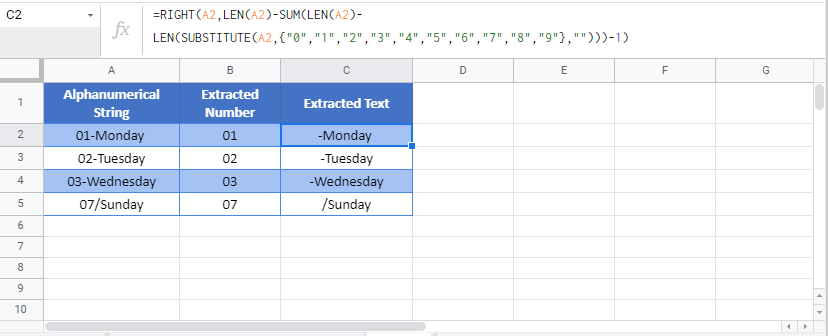### Excel Practice Worksheet

Practice Excel functions and formulas with our 100% free practice worksheets!